For K means clustering algorithm, I will be using Credit Cards Dataset for Clustering from Kaggle.

In :
import numpy as np
import pandas as pd

import matplotlib.pyplot as plt

%matplotlib inline


#### Data preprocessing¶

In :
credit_data = pd.read_csv('../data/CC GENERAL.csv')

In :
credit_data.head()

Out:
CUST_ID BALANCE BALANCE_FREQUENCY PURCHASES ONEOFF_PURCHASES INSTALLMENTS_PURCHASES CASH_ADVANCE PURCHASES_FREQUENCY ONEOFF_PURCHASES_FREQUENCY PURCHASES_INSTALLMENTS_FREQUENCY CASH_ADVANCE_FREQUENCY CASH_ADVANCE_TRX PURCHASES_TRX CREDIT_LIMIT PAYMENTS MINIMUM_PAYMENTS PRC_FULL_PAYMENT TENURE
0 C10001 40.900749 0.818182 95.40 0.00 95.4 0.000000 0.166667 0.000000 0.083333 0.000000 0 2 1000.0 201.802084 139.509787 0.000000 12
1 C10002 3202.467416 0.909091 0.00 0.00 0.0 6442.945483 0.000000 0.000000 0.000000 0.250000 4 0 7000.0 4103.032597 1072.340217 0.222222 12
2 C10003 2495.148862 1.000000 773.17 773.17 0.0 0.000000 1.000000 1.000000 0.000000 0.000000 0 12 7500.0 622.066742 627.284787 0.000000 12
3 C10004 1666.670542 0.636364 1499.00 1499.00 0.0 205.788017 0.083333 0.083333 0.000000 0.083333 1 1 7500.0 0.000000 NaN 0.000000 12
4 C10005 817.714335 1.000000 16.00 16.00 0.0 0.000000 0.083333 0.083333 0.000000 0.000000 0 1 1200.0 678.334763 244.791237 0.000000 12
##### A. Check for missing data¶
In :
credit_data.isna().sum()

Out:
CUST_ID                               0
BALANCE                               0
BALANCE_FREQUENCY                     0
PURCHASES                             0
ONEOFF_PURCHASES                      0
INSTALLMENTS_PURCHASES                0
PURCHASES_FREQUENCY                   0
ONEOFF_PURCHASES_FREQUENCY            0
PURCHASES_INSTALLMENTS_FREQUENCY      0
PURCHASES_TRX                         0
CREDIT_LIMIT                          1
PAYMENTS                              0
MINIMUM_PAYMENTS                    313
PRC_FULL_PAYMENT                      0
TENURE                                0
dtype: int64

We can see that some missing values in column MINIMUM_PAYMENTS column. Since we are focusing on algorithm aspect in this tutorial, I will simply remove entries having 'NaN' value.

##### B. Remove 'NaN' entries¶
In :
credit_data = credit_data.dropna(how='any')

##### C. Remove nonrelevant column/feature¶
In :
# Customer ID does not bear any meaning to build cluster. So, let's remove it.
credit_data.drop("CUST_ID", axis=1, inplace=True)

Sijan Bhandari onSijan Bhandari on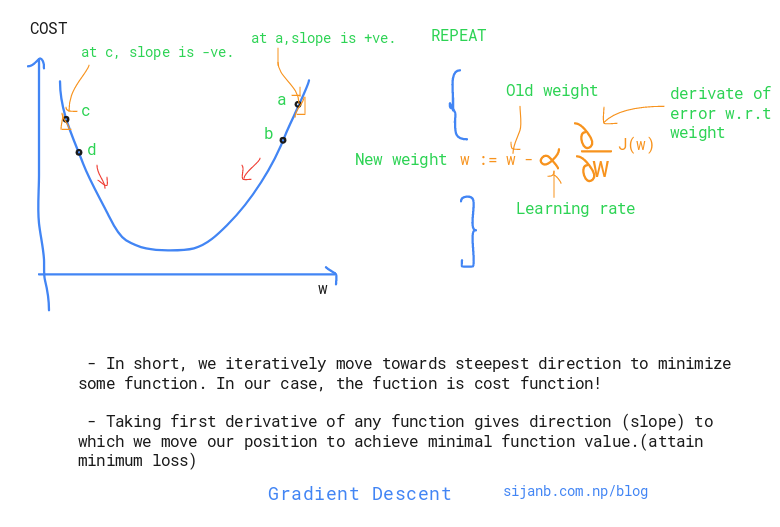Before jumping to Gradient Descent, let's be clear about difference between backpropagation and gradient descent. Comparing things make it easier to learn !

##### Backpropagation :¶

Backpropagation is an efficient way of calculating gradients using chain rule.

Gradient Descent is an optimization algorithm which is used in different machine learning algorithms to find parameters/combination of parameters which mimimizes the loss function.

** In case of neural network, we use backpropagation to calculate the gradient of loss function w.r.t to weights. Weights are the parameters of neural network.

** In case of linear regression, coefficients are the parameters!.

** Many machine learning algorithms are convex problems, so using gradient descent to get extrema makes more sense. For example, if you remember the solution of linear regression :

$\beta = (X^T X)^{-1} X^T y$

Here, we can get the analytical solution by simply solving above equations. But, inverse calculation has $O(N^3)$ complexity. It will be worst if our data size increases.

Sijan Bhandari on

Network has been taken as a tool for describing complex systems or interactions around us. Few prominent complex systems are:

1. Our society where almost 7 billions individuals exist/ and the interactions between them in one or other ways.

2. Genes in our body, interactions between gene molecules ( Protein-Protein interaction networks)

Peoply usually visualize the network to see cluter/ densely linked clusters and try to analyze, predict relation between nodes, figure out similarity between nodes in the network.

Figuring out the central nodes/vertices is also an important network analysis process because centrality measures :

        a. Existing influence of a node on other nodes
b. Information flow in and out from a node or towards it
c. Finding node/s which is/are acting as bridge between two different/big groups
Sijan Bhandari on

In any document, the frequency of occurrence of terms is taken as an important measure of score for that document (Term Frequency). For example : a document has total 100 words, and 30 words are 'mountains', we ,without hesitation, say that this document is talking about 'Mountains'.

But, if we only include most frequent word as our score metric, we will eventually loose the actual relevancy score of the document. Since same word could exist in number of documents and it's just frequent occurrence without adding much meaning to current context. In the above example : Suppose, there are two documents talking about 'Mt. Everest'. We obviously know that there will be higher occurrence of word 'Mountains'. But, if we use 'Term Frequecy (tf)' alone, term 'Mountains' will get highest weight rather than term 'Everest'. It's not fair. And, Inverse-Document-Frequency will tackle it.

#### Term Frequency (TF) / Normalized Term Frequency (nTF):¶

It simply measures the frequency/occurent of a term in a document. So, it gives equal important to all terms. Longer document could have large number of terms than smaller documents, so better to normalize this metric by dividing with total number of terms in the document. We also

#### Applications:¶

1. Summarizing a document by extracting keywords.
2. Comparing two documents (similary/ relevancy check)
3. Search query to documents matching for building query results for search engine
4. Weighting 'terms' in the document.

#### Inverse Document Frequency (IDF):¶

It gives the importance to more relevant/significant term in the document. It tries to lower the weights to terms having less importance. And, rare terms will get significant weights.

#### TF-IDF:¶

It tries to prioritize the terms based on their occurrence and uniqueness.

Suppose, I have two documents in my corpus and I want to give tf-idf weighting to the terms.

##### Document II : 'Nepal is a landlocked Country'¶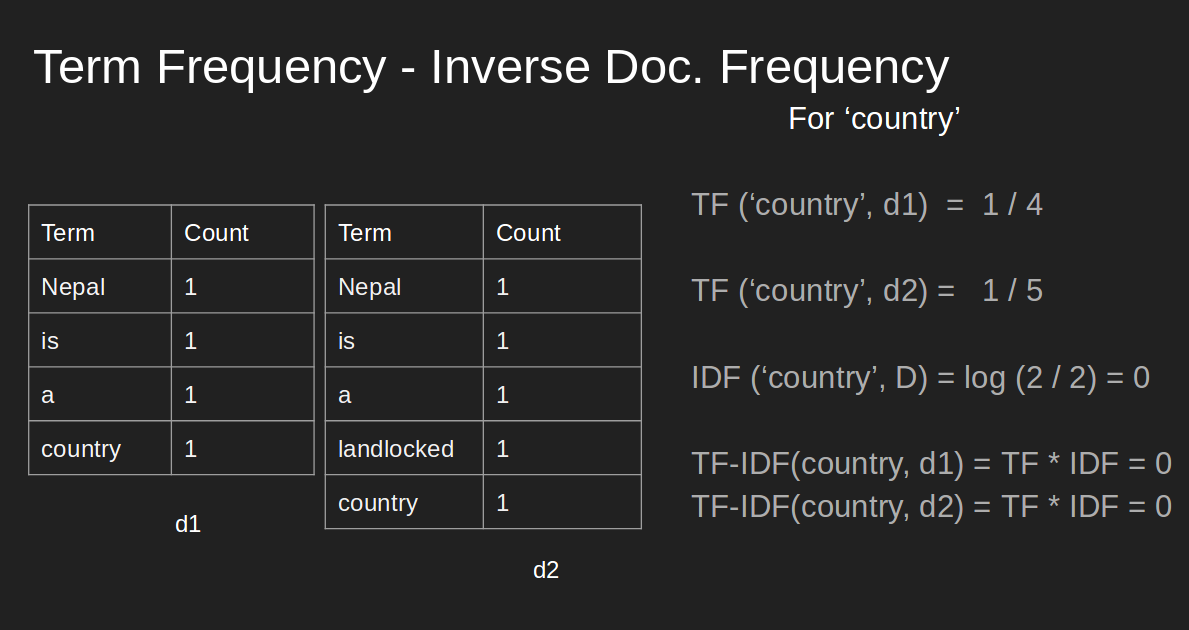We can see that, although, term 'country' has prominent occurrence, 'tf-idf' gives priority to word 'landlocked' and it carries more information about the document.

#### NOTE 1 :¶

These weights are eventually used for vector-space model, where each term represents the axes, and document are the vectors on that space. Since 'tf-idf' value is zero (as shown above)' this representation is very sparse.

#### NOTE 2 :¶

Suppose, we are building a search engine system. The query is also converted into vector in vector-space model and compare with documents (NOTE 1) to get the similarity between them.

In [ ]:


Sijan Bhandari on

In this post, we will see how we can train support vector machines using stochastic gradient descent (SGD). Before jumping to the algorithm, we need to know why subgradients?

Here is a brief summary of the 0-1 loss and hinge loss:But, why don't we use 0-1 loss ?? The obvious reason is it is not convex. Another factor could be it's reaction to small changes in parameters. You can see from the graph, if you change (w,b) the loss will flip to either 0 or 1 very fast without acknowledging the in between values. The hinge loss,on the other hand, has smooth change until it reaches '1' along x-axis.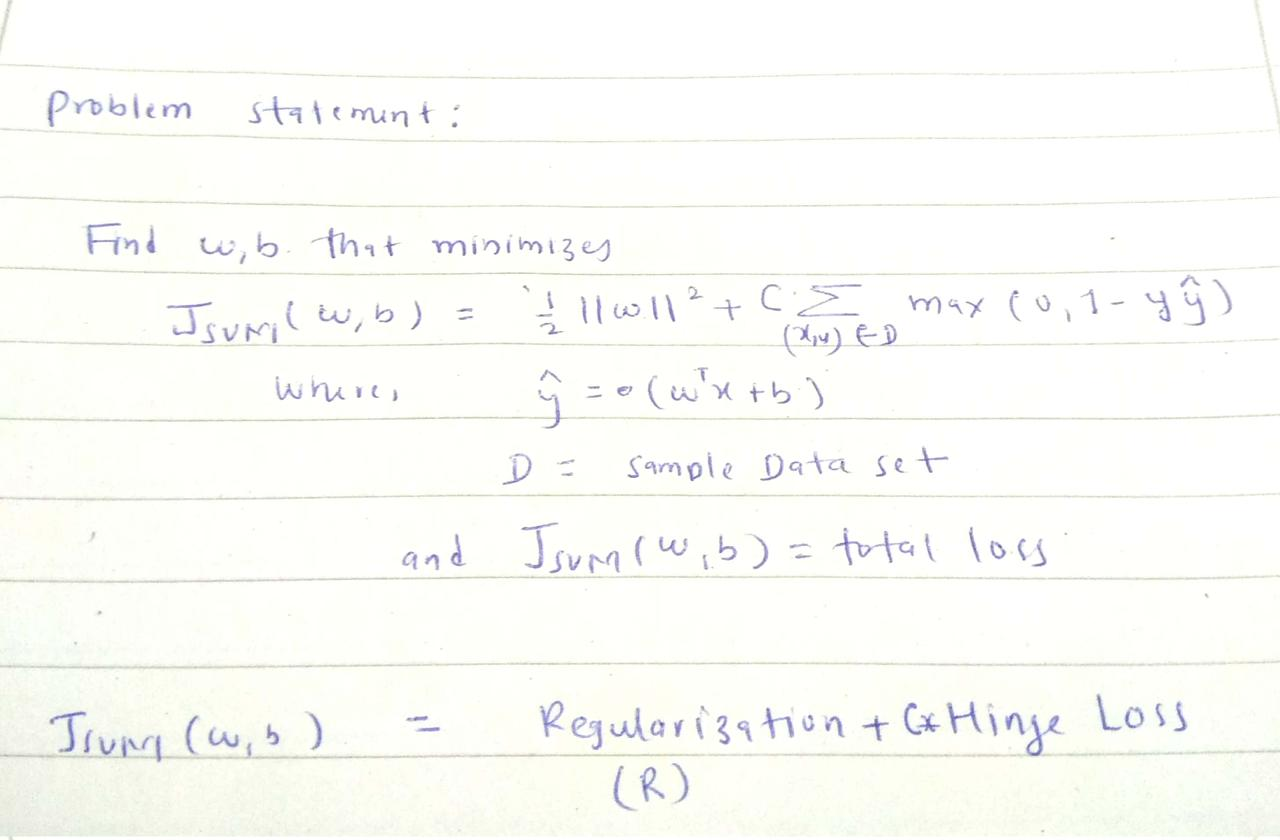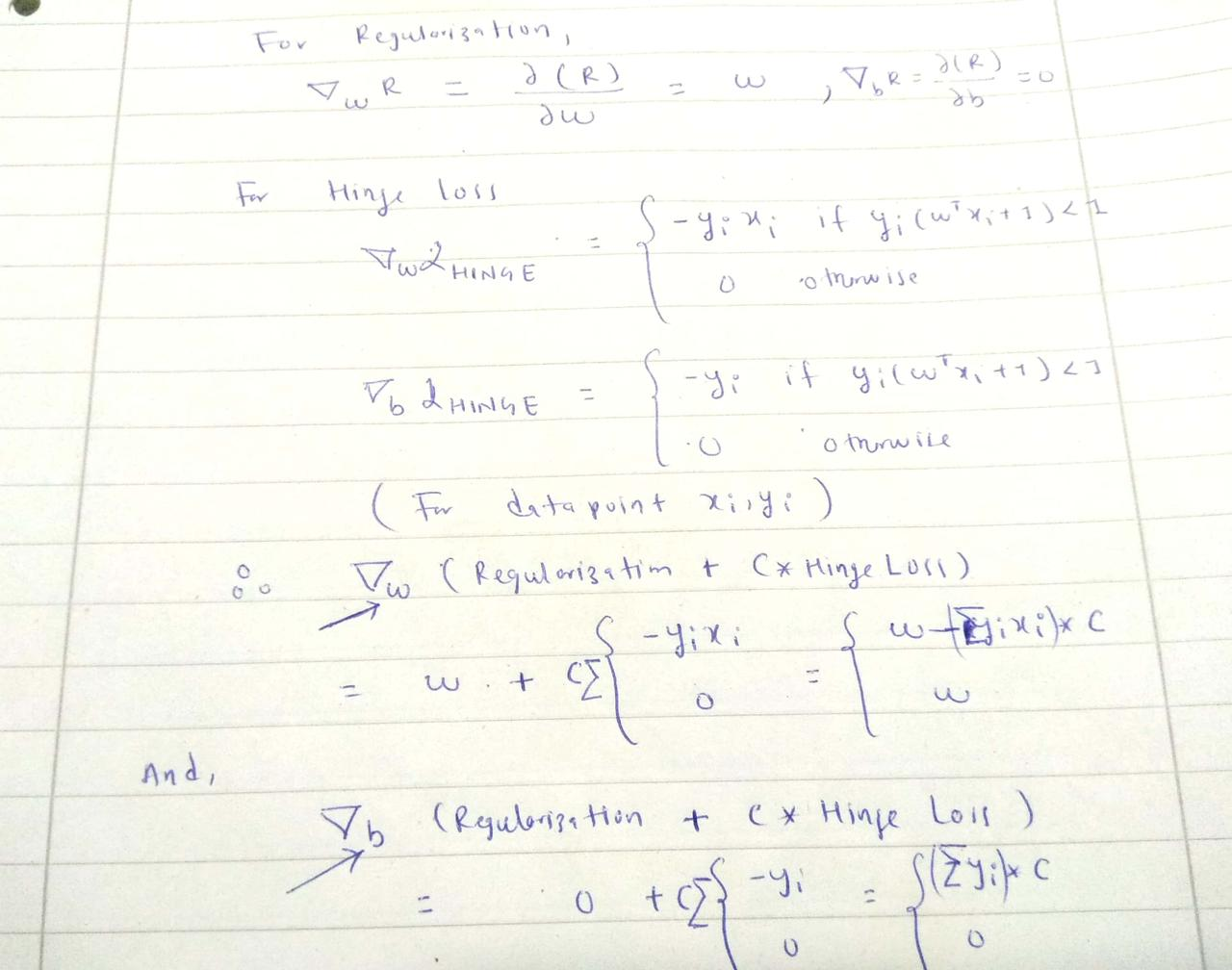In :
import pandas as pd
import numpy as np

In :
def add_regularization(w, subgradient_w):
"""
The total loss :( 1/2 * ||w||^2 + Hingle_loss) has w term to be added after getting subgradient of 'w'

total_w = regularization_term + subgradient_term
i.e total_w = w + C *  ∑ (-y*x)

"""
return w + subgradient_w

In :
def subgradients(x, y, w, b, C):
"""
:x: inputs [[x1,x2], [x2,x2],...]
:y: labels [1, -1,...]
:w: initial w
:b: initial b

"""

# sum over all subgradients of hinge loss for a given samples x,y
for x_i, y_i in zip(x,y):
f_xi = np.dot(w.T, x_i) + b

decision_value = y_i * f_xi

if decision_value < 1:
subgrad_w += - y_i*x_i
subgrad_b += -1 * y_i
else:

# multiply by C after summation of all subgradients for a given samples of x,y

In :
def stochastic_subgrad_descent(data, initial_values, B, C, T=1):
"""
:data: Pandas data frame
:initial_values: initialization for w and b
:B: sample size for random data selection
:C: hyperparameter, tradeoff between hard margin and hinge loss
:T: # of iterations

"""
w, b = initial_values
for t in range(1, T+1):

# randomly select B data points
training_sample = data.sample(B)

# set learning rate
learning_rate = 1/t

# prepare inputs in the form [[h1, w1], [h2, w2], ....]
x = training_sample[['height', 'weight']].values

# prepare labels in the form [1, -1, 1, 1, - 1 ......]
y = training_sample['gender'].values

# update weights
w = w - learning_rate * sub_grads

# update bias
b = b - learning_rate * sub_grads
return (w,b)

In :
data = pd.DataFrame()

data['height'] = np.random.randint(160, 190, 100)
data['weight'] = np.random.randint(50, 90, 100)

data['gender'] = [-1 if value < 5 else 1 for value in np.random.randint(0,10, 100)]


Out:
height weight gender
0 167 82 1
1 183 57 -1
2 184 50 -1
3 178 53 -1
4 166 72 1
In :
initial_weights = np.array([-2, -3])
initial_bias = 12

initial_values = (initial_weights, initial_bias)

In :
w,b = stochastic_subgrad_descent(data, initial_values, 20, 1, 1000)

In :
w,b

Out:
(array([-0.798,  4.648]), 14.891692339980313)
In [ ]:


Sijan Bhandari on

Support vector machine (SVM) is a supervised machine learning algorithm which is considered effective tool for both classification and regression problem.

In a simple word, SVM tries to find a linearly separable hyperplane in order to separate members of one class from another. If SVM can not find the hyperplane for a given data set, it applies non-linear mapping to the training data and transform them to higher dimension where it searches for the optimal hyperplane. The SVM algorithm uses support vectors and margins in order to draw these hyperplanes in the training data.

Since it has ability to understand the complex relation in input data by applying nonlinear mapping, it has high accuracy compare to other supervised classification algorithms (kNN, NCC..)

People have been using SVM for different applications like : text data classification, image data(handwritten) recognition and more.

Intuition:

Let us see a linearly separable problem as shown in the diagram below.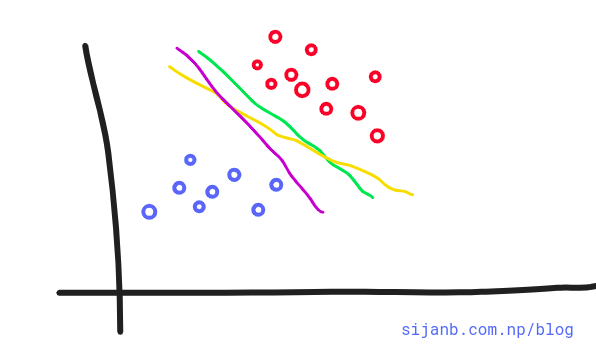In the given diagram, we can draw infinite lines that can separate data into two different classes.

Can you decide Which of the lines (pink, yellow, green) better suits our problem? If you look closely, the green line is close to red dots and any minor change in data point may caues this point to fall into other class. On the other hand, Pink line is close to blue dots and similarly, a minor twist on data are prone to change the class of new point to other side and may misclassified.

But, the yellow line looks more stable, in the sense, it is far from data points on either side and not susceptible to the small changes in data observations.

Support vector machines help us to make a decision on this problem. If you think 'yellow' line in the figure is the optimal hyperplane for this problem, we can take this as an intuition that an optimal choice from the number of choices of hyperplanes is the one which is farthest from our training data points.

We can compute the perpendicular distances from all our training points to all possible hyperplanes and choose the one which has largest minimum distance to the training examples. The minimum distance between hyperplane and the observation point is called margin.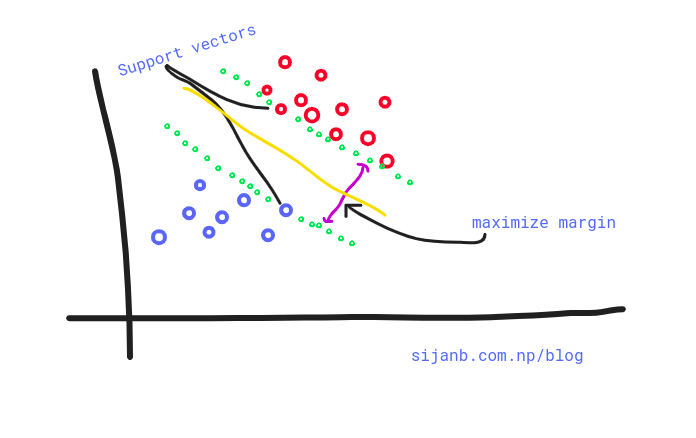In summary, SVMs maximize the margin around our separating hyperplane. And, The idea is to draw a margin of some width around the separating hyperplane, up to the nearest point.

The training data that falls exactly on the boundaries of the margin are called

the support vectors. If these points shifted on any direction, they also influence the hyperplanes passing through the margin points and they also shifted. It is important to notice that support vectors are critical data points on our training set since they are the one defining margin boundaries.

It is important to note that the complexity of SVM is characterized by the number of support vectors, rather than the dimension of the feature space. That is the reason SVM has a comparatively less tendency to overfit.

#### Support vectors "support the plane" and are hence called Support Vectors.¶

Upto now, we are trying to find the hard-margin for our hyperplane. But, as shown in the diagram below, a single point can have high influence on our boundary. So hard margin SVM are sensitive to outliers, giving overfitted model.What about we relax our hard margin condition? where, a small amount of data points are allowed to cross the margins/boundaries .i.e data points can be on incorrect side as well. This approach is called soft-margin. Soft in the sense that few data points can violate the margin condition. The softness is measured by slack variable; which control the position of training data points relative to the margins and our hyperplane. The ultimate goal is, our SVM tries to maximize the soft margin.In [ ]:


Sijan Bhandari on

The Nearest centroid classifier (NCC) says that we should classify a data point to a class whose centroid is closest to this data point.

The algorithms follows:

Suppose $c_l$ represents the set of indices which belong to class l. And n = |$c_l$|

##### 1.Training step :¶

We compute the centroids(CTs) for each of the classes as:

$CT_l$ = $\frac{1}{n} \sum_{i \in c_l} x_i$

##### 2.Prediction step :¶

a. Given a new data point $x_{new}$, compute the distance between $x_{new}$ and each centroids as

distance : $|| x_{new} -$CT_l$||_2$ (Euclidean distance)

b. Assign the class to this new point which has minimum distance value.

Let us taken an example. We have to classify fruits into two classes : Apple and Orange, based on their height and width.

Our inputs (x) are :

x1=[5,6], x2=[5,7], x3=[4,3], x4=[5,7], x5=[6,4] and corrresponding labels (y) are

𝑦1='AP' 𝑦2='AP' 𝑦3='AP' 𝑦4='ORG' 𝑦5='ORG'

Here $x_i$ = [width, height] , 'AP' = 'Apple', 'ORG' = 'Orange'.

Now, centroids for two classes are :

$CT_{AP}$ = $\frac{1}{3} (5 + 5 + 4, 6 + 7 + 3)$ = ($\frac{14}{3}, \frac{16}{3}$)

$CT_{ORG}$ = $\frac{1}{2} (5 + 6, 7 + 4)$ = ($\frac{11}{2}, \frac{11}{2}$)

Suppose, you got a new test data point : (3, 7) i.e $x_{new}$, and you want to classify this point. We can calculate the distance between new point and our centroids as:

$||x_{new} - CT_{AP} ||$ = || (3,7) - ($\frac{14}{3}, \frac{16}{3}$) || = 2.357

$||x_{new} - CT_{ORG} ||$ = || (3,7) - ($\frac{11}{2}, \frac{11}{2}$) || = 2.915

Here, the new data point is classified as 'Apple' as the new data point is closest to the centroid of data points that belong to class 'Apple'

In [ ]:


Sijan Bhandari on

K-Nearest Neighbors (k-NN) is one of the classification algorithms in Machine learing. Since, K-NN simply memories or stores the rules in memory, we can also say that it does not follow actual machine learning paradigm.

Before going to implementation, let us build some standard notation so that it is easier to follow the code in the implementation section (next post):

          X_training = given training data
Y = labels for your given training data
X_test = new data (For which we need to predict the labels)



The whole algorithm can be divided into three major steps:

1. Finding most nearest neighbors (similar data instances) from your training data for a given test data (X_test)

Let's say we have total 20 training data, and you find out 4 training instances as nearest neighbors for
one of your test data
2. Once you get the nearest neighbors from your training data, collect all the labels of your selected training data

In our case, we have 4 training instances as nearest neighbors. So, we will collect labels for all
these 4 training data. 
3. Finally, predict the label of your test data (X_test) based on majority count.

      In our case, suppose 3 out of 4 training instances have the same label. Then, the majority count
will assign the label to new data point

The K defines the number of neighbors that the algorithm will collect for making the prediction of your new data.

###### Example¶

Suppose, we have a data set of a students with features : weight and height and labels as "Asian, European, American" as follows:

In :
from IPython.display import HTML, display
import tabulate
table = [["Height(cm)","Weight(kg)","Class"],
[169, 60, 'Asian'],
[171, 59, 'Asian'],
[172, 70, 'European'],
[179, 69, 'European'],
[170, 75, 'Asian'],
[175, 80, 'American'],
[176, 79, 'American'],
[180, 71, 'European'],
[171, 76, 'American'],

]
display(HTML(tabulate.tabulate(table, tablefmt='html')))

 Height(cm) Weight(kg) Class 169 60 Asian 171 59 Asian 172 70 European 179 69 European 170 75 Asian 175 80 American 176 79 American 180 71 European 171 76 American

Now, suppose new student enters the class with feature

     Height : 168
Weight : 65



Which class this new student belongs to?

Now, let us use some distance measure between this new point and all the training data points as follows:

sqrt((169-168)^2 + (60-65)^2) = 5.09

In :
import math
def distance(p1, p2):
sq_distance = (p1 - p2)**2 + (p1 - p2)**2
return math.ceil(sq_distance**(1/2))

In :
table = [["Height(cm)","Weight(kg)","Class", "Distance"],
[169, 60, 'Asian', distance([169, 60], [168, 65])],
[171, 59, 'Asian', distance([171, 59], [168, 65])],
[172, 70, 'European', distance([172, 70], [168, 65])],
[179, 69, 'European', distance([179, 69], [168, 65])],
[170, 75, 'Asian', distance([170, 72], [168, 65])],
[175, 80, 'American', distance([175, 80], [168, 65])],
[176, 79, 'American', distance([176, 79], [168, 65])],
[180, 71, 'European', distance([180, 71], [168, 65])],
[171, 76, 'American', distance([171, 76], [168, 65])],

]
display(HTML(tabulate.tabulate(table, tablefmt='html')))

 Height(cm) Weight(kg) Class Distance 169 60 Asian 6 171 59 Asian 7 172 70 European 7 179 69 European 12 170 75 Asian 8 175 80 American 17 176 79 American 17 180 71 European 14 171 76 American 12

From the result table, let's select k=3, neighbors having minimal distance(nearest points)

 a. 169     60  Asian         6
b. 171     59  Asian         7
c. 172     70  European        7 

So all possible labels in our final result is : ['Asian', 'Asian', 'European']

With majority vote, we can classify the new student as 'Asian'

In [ ]:


Sijan Bhandari on

Let me start with an example. Suppose you have built a ML model under supervised learning which can detect faces from your family members. You simply feed all the family photos to your model. And, at the end, you would like to know how much your model learnt from your training process.After training your model, you will find a function that maps input (image) to output labels(family members). If we represent your ML model with a function 'f',we can write this process as:

  y = f(x),
where  x = input  (your family photos)
y = output (model prediction) (predictiong whether the photo is your father, mother or sister)



Suppose, you use 50 images for your training process and use the same images to see whether the model can predict the label correctly or not. If you found that 10 out of 50 images are not predicted well, you can say : 20% training error. If you think about the intuition behind this calculation, it is saying that 'the model is not doing well with the training data itself'. That means it underfits the data. It does not want to carry all the information available in your data.

*NOTE: If your model 'f' is a linear model than it will be really hard for your model to classify the photos using linear function.

Sijan Bhandari on